# Data Visualization

## Methods of Data Visualizatons and their Uses

Data visualization refers to presenting data in a pictorial or graphical format using different graphs such as histogram, polygon, line chart and bar chart.

### Histogram

A histogram is a graphical representation of the data contained in the frequency distribution. It is a bar chart of data that groups data into intervals. The intervals should capture all the data points and also be non-overlapping. It is constructed by plotting the intervals on the horizontal axis and the absolute frequencies on the vertical axis.

For a histogram with equal size intervals, a rectangle should be erected over the interval, with its height being proportional to the absolute frequency. If intervals are unequal in size, the erected rectangle has an area proportional to the absolute frequency of that particular interval. In such a case, we would have the vertical axis labeled as ‘density’ instead of frequency. There should be no space between bars to indicate that the intervals are continuous.

#### Example 1: Histogram

Consider the previous example of the returns offered by a stock. To bring you up to speed, these were the intervals and the corresponding frequencies:

$$\begin{array}{c|c|c} \text { Interval } & \text { Tally } & \text { Frequency } \\ \hline-30 \% \leq \mathrm{R}_{\mathrm{t}} \leq-20 \% & \text { II } & 2 \\ -20 \% \leq \mathrm{R}_{\mathrm{t}} \leq-10 \% & \text { I } & 1 \\ -10 \% \leq \mathrm{R}_{t} \leq 0 \% & \text { III } & 3 \\ 0 \% \leq \mathrm{R}_{t} \leq 10 \% & \text { IIIII } & 6 \\ 10 \% \leq \mathrm{R}_{t} \leq 20 \% & \text { IIIIII } & 7 \\ 20 \% \leq \mathrm{R}_{t} \leq 30 \% & \text { IIII } & 5\\ 30 \% \leq \mathrm{R}_{t} \leq 40 \% & \text { I } & 1 \\ \text { Total } & & 25 & =25 / 25=100 \% \end{array}$$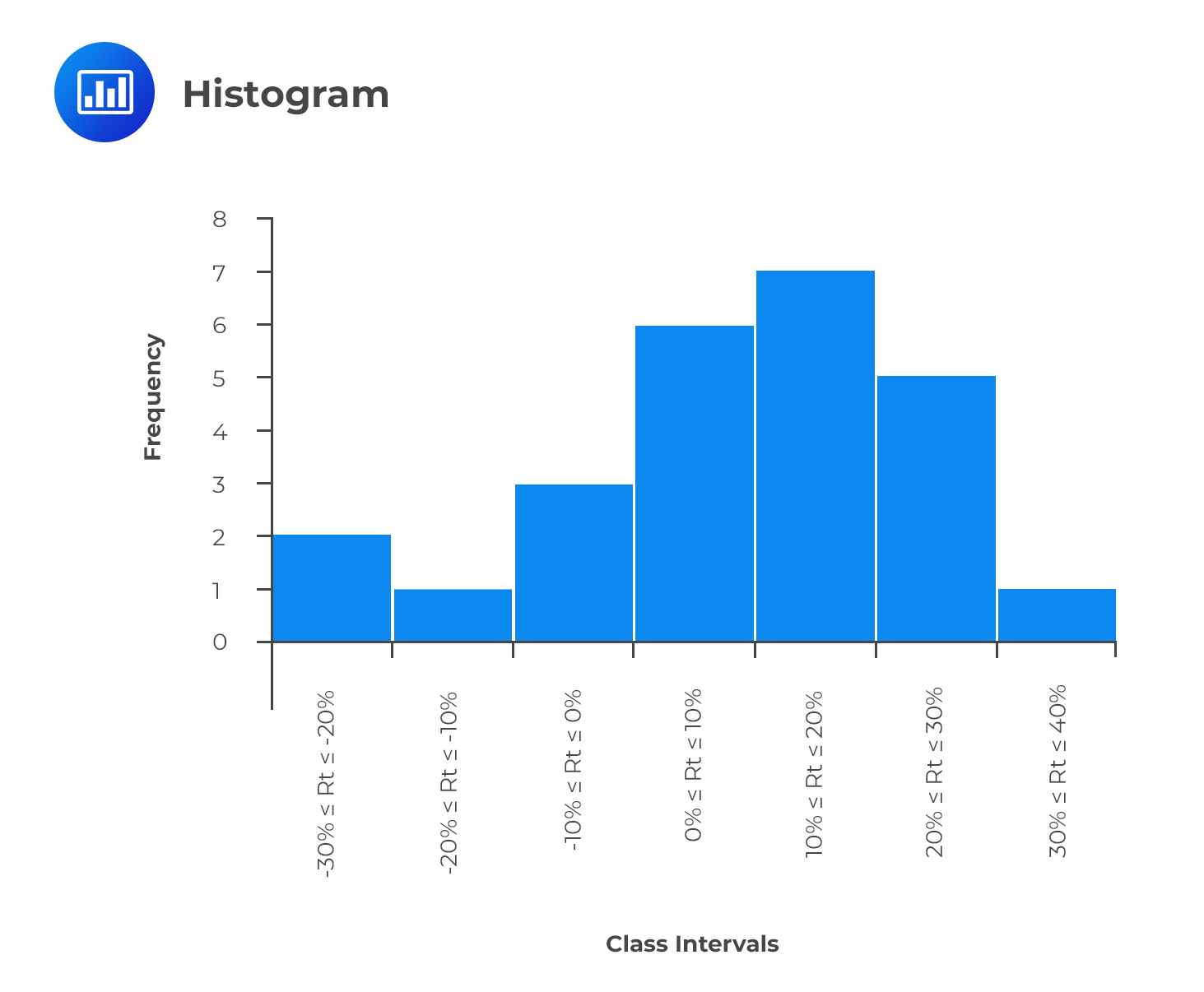As mentioned, histograms can also be created with relative frequencies—the choice of using absolute versus relative frequency depends on the question being answered. An absolute frequency histogram best answers the question of how many items are in each bin. In contrast, a relative frequency histogram gives the proportion or percentage of the total observations in each bin.

### Frequescy Polygon

Frequency polygon is used to represent the distribution of data graphically. However, it has a major difference when compared to the histogram. Instead of having the class intervals on the horizontal axis clearly showing their upper and lower limits, a frequency polygon uses the midpoints of the class intervals where:

$$\text{Midpoint of a class interval}= \text{Lower limit}+\frac{\text{(Upper limit-Lower limit}}{2}$$

The vertical axis features the absolute frequencies which are then joined using straight lines and markers.

#### Example 2: Frequency Polygon

Going back to the stock return data, we could come up with a frequency polygon.

To come up with the midpoints, we use the formula above. As an example, the midpoint of the interval -30% ≤ Rt ≤ -20% is:

$$\text{Midpoint} = -30 + \cfrac {(-20 – – 30)}{2} = -25$$

We can calculate the midpoints for the other intervals in a similar manner. The final frequency polygon should look like this: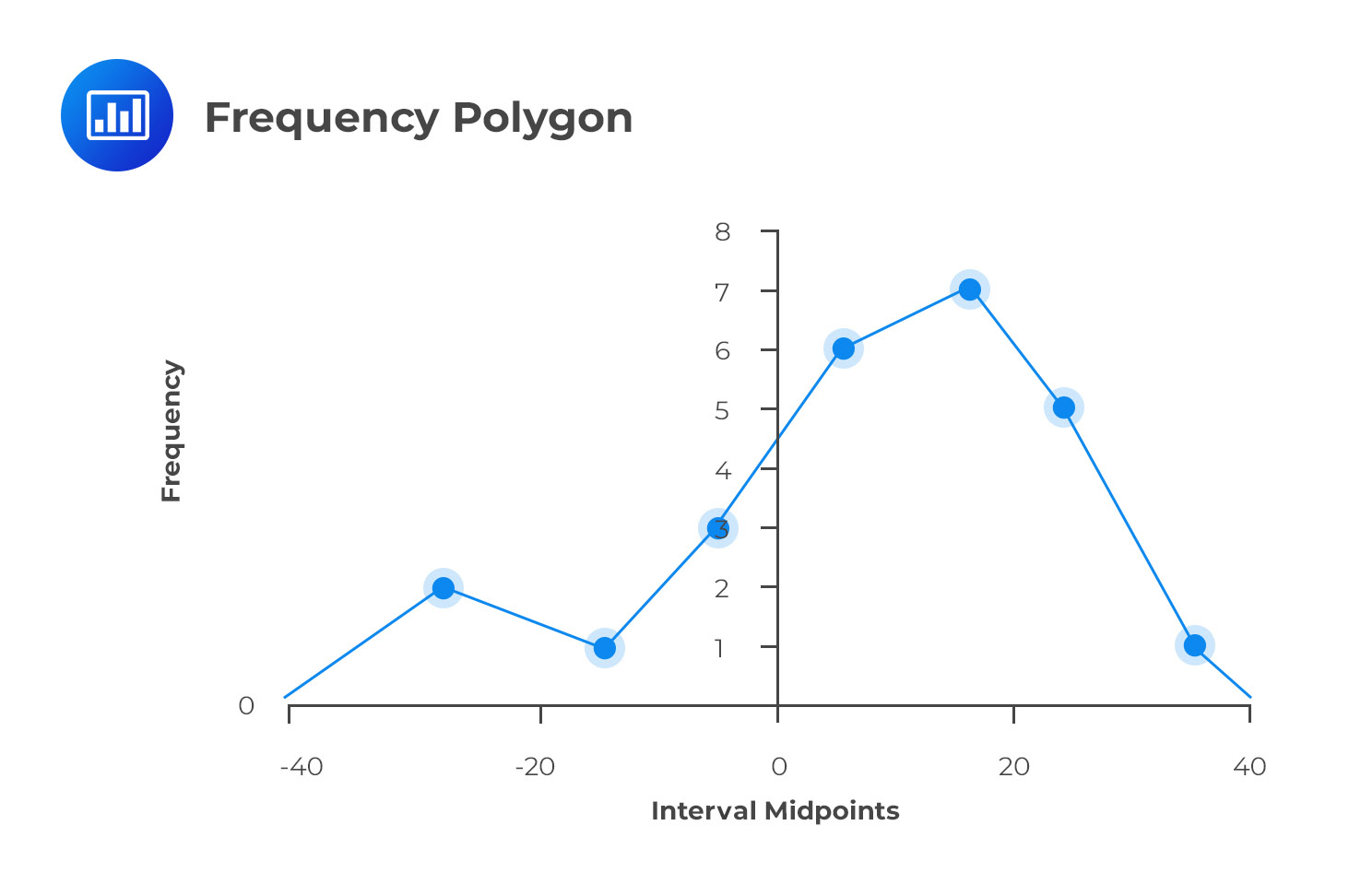The frequency polygon is important because it shows the shape of a distribution of data. It can also be very useful when comparing two sets of data side-by-side. Note that the endpoints touch the X-axis. The vertical scale can also be positioned at the left margin.

A cumulative frequency distribution graph can plot the cumulative frequency or relative frequency against the upper interval limit. The cumulative frequency distribution allows us to see how many or what percent of the observations lie below a certain value. The figure below is an example of a cumulative frequency distribution.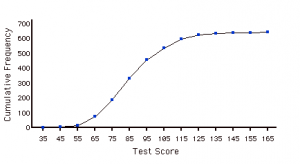The change in the cumulative relative frequency as we move from one interval to the next represents the interval’s relative frequency. When the slope of cumulative frequency distribution is steep, it indicates that these frequencies are large. It is pertinent to note that the slope of the cumulative absolute distribution at any particular interval is proportional to the number of observations in that interval.

### Bar Chart

Bar chart is used to plot the frequency distribution of categorical data. In a bar chart, each bar represents a distinct category, while the height of a bar is proportional to the frequency of the corresponding category. Bar charts can be vertical or horizontal.

• In a vertical (horizontal) bar chart, the y-axis (x-axis) represents the absolute Frequency or the relative Frequency. In contrast, the x-axis (y-axis) represents the mutually exclusive categories to be compared rather than bins that group numerical data (unlike histogram).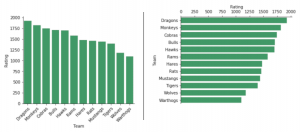### Pareto Chart

A bar chart where the categories are ordered by frequency in descending order and a line displaying cumulative relative frequency is known as a Pareto Chart. This chart is used to highlight dominant categories or the most important groups.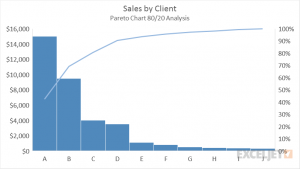### Grouped Bar Chart

A grouped bar chart (also known as a clustered bar chart) plots two categorical variables to represent their joint frequencies.

### Stacked Bar Chart

Stack bar chart is also used to present the joint frequency distribution of two cat­egorical variables. In a vertical stacked bar chart, the bars representing the sub-groups are placed on top of each other to form a single bar. Each subsection of the bar is shown in a different color to represent the contribution of each sub-group, and the overall height of the stacked bar represents the marginal frequency for the category.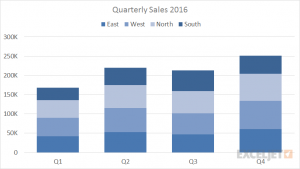### Tree-Map

A treemap chart displays hierarchical data. A rectangular shape represents each item on a treemap, where smaller rectangles represent the sub-groups. The color and size of rectangles are typically correlated with the tree structure. The area of each rectangle is proportional to the value of the corresponding group.

The treemap shown below depicts the revenue of different companies in the food sector. We can observe that ABC Company has the highest revenue in the sector, represented by the rectangle with the largest area.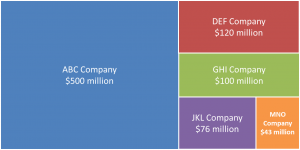Additional dimensions can be added by displaying a set of nested rectangles – as shown below.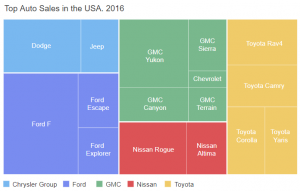### Word Cloud

Word Clouds (also known as a tag clouds) are a visual representation of textual data. The size of each specific word is proportional to the frequency of words within a given body of text. We can use a different color to convey different sentiments. For example, profit can be displayed using green color while loss can be displayed using red color.  Word cloud is generally used to display unstructured data.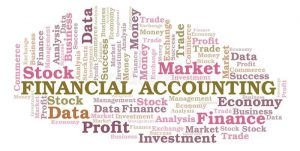### Line Chart

A line chart is used to display the change of data series over time. Note that the frequency polygon and the cumulative frequency distribution chart are also line charts, representing data frequency distributions.

In a line chart, the x-axis represents period (say years), and the y-axis represents data that we want to plot (say real GDP growth rate).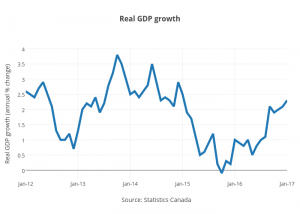A line chart can be drawn using more than one set of data points, which can be used for making comparisons.

### Bubble Line Chart

In a bubble line chart, data points are represented by varying-sized bubbles to represent a third dimension of the data. These bubbles can be of different colors to represent additional information, i.e., red bubbles for negative values and green bubbles for positive values.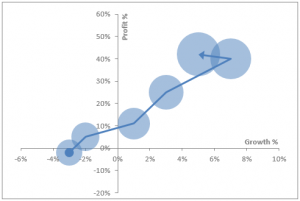### Scatter Plot

A scatterplot is a type of graph that shows the relationship between two numerical variables. It helps in displaying and understanding potential relationships between two variables. In a scatterplot, one variable is plotted on the x-axis, and another variable is plotted on the y-axis.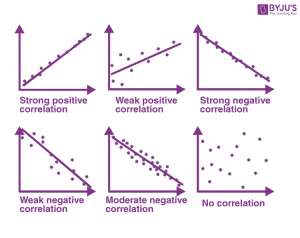As shown above, a positive (negative) slope for the line of data points indicates a positive (negative) relation between the two variables. The strength of the relationship between variables can be determined based on how closely the data points are clustered around the line. Tight (loose) clustering indicates a potentially stronger (weaker) relationship. Further, data points located toward the ends of each axis represent the maximum or minimum values (i.e., outliers).

### Scatter Plot Matrix

A scatter plot matrix is a grid of scatter plots used to visualize bivariate relationships between combinations of variables.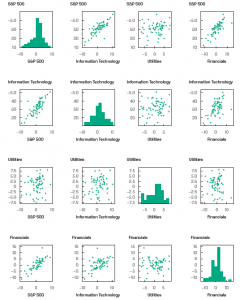### Heat Map

A heatmap is a graphical representation of data that uses a system of color-coding to represent different values. More intense color is displayed as the marks “heat up” due to their higher values or density of records. Heatmap can be used to display frequency distributions and visualize the degree of correlation among different variables.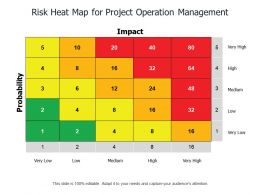### Question

Which of the following most likely represents the graph which is drawn by connecting successive mid-points in a histogram by straight lines:

1. Line graph.
2. Frequency curve
3. Frequency polygon

Solution:

C is correct. When successive mid-points in a histogram are connected by straight lines, the graph is called a frequency polygon.

Reading 2 LOS 2e: Describe ways that data may be visualized and evaluate the uses of specific visualizations.

Quantitative Methods – Learning Sessions

Shop CFA® Exam Prep

Offered by AnalystPrepLevel I
Level II
Level III
All Three Levels
Featured Shop FRM® Exam PrepFRM Part I
FRM Part II
FRM Part I & Part II
Learn with Us

Subscribe to our newsletter and keep up with the latest and greatest tips for success
Shop Actuarial Exams PrepExam P (Probability)
Exam FM (Financial Mathematics)
Exams P & FM
Shop GMAT® Exam PrepComplete CourseSergio Torrico
2021-07-23
Excelente para el FRM 2 Escribo esta revisión en español para los hispanohablantes, soy de Bolivia, y utilicé AnalystPrep para dudas y consultas sobre mi preparación para el FRM nivel 2 (lo tomé una sola vez y aprobé muy bien), siempre tuve un soporte claro, directo y rápido, el material sale rápido cuando hay cambios en el temario de GARP, y los ejercicios y exámenes son muy útiles para practicar.diana
2021-07-17
So helpful. I have been using the videos to prepare for the CFA Level II exam. The videos signpost the reading contents, explain the concepts and provide additional context for specific concepts. The fun light-hearted analogies are also a welcome break to some very dry content. I usually watch the videos before going into more in-depth reading and they are a good way to avoid being overwhelmed by the sheer volume of content when you look at the readings.Kriti Dhawan
2021-07-16
A great curriculum provider. James sir explains the concept so well that rather than memorising it, you tend to intuitively understand and absorb them. Thank you ! Grateful I saw this at the right time for my CFA prep.nikhil kumar
2021-06-28
Very well explained and gives a great insight about topics in a very short time. Glad to have found Professor Forjan's lectures.Marwan
2021-06-22
Great support throughout the course by the team, did not feel neglectedBenjamin anonymous
2021-05-10
I loved using AnalystPrep for FRM. QBank is huge, videos are great. Would recommend to a friendDaniel Glyn
2021-03-24
I have finished my FRM1 thanks to AnalystPrep. And now using AnalystPrep for my FRM2 preparation. Professor Forjan is brilliant. He gives such good explanations and analogies. And more than anything makes learning fun. A big thank you to Analystprep and Professor Forjan. 5 stars all the way!michael walshe
2021-03-18
Professor James' videos are excellent for understanding the underlying theories behind financial engineering / financial analysis. The AnalystPrep videos were better than any of the others that I searched through on YouTube for providing a clear explanation of some concepts, such as Portfolio theory, CAPM, and Arbitrage Pricing theory. Watching these cleared up many of the unclarities I had in my head. Highly recommended.